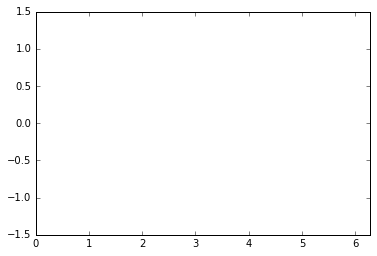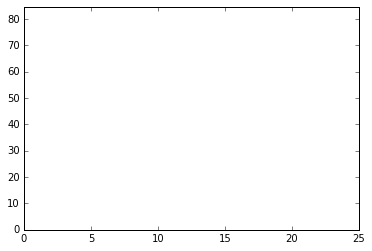# Animation and Interactive Demonstration

This notebook is to demonstrate animation and interactive elements in python + Jupyter.

## Animation Background

The animation is a part of matplotlib. For the animation, we create a function that generates each frame. This function is passed to the matplotlib.animation class creator FuncAnimation to generate the animation. We can then display that animation in the notebook and/or save it to a file. Some good resources to understand animation using matplotlib are:

## Interactive Elements Background

The imports from ipywidgets allow us to create interactive sliders and other elements in the notebook. Some good information on this is at

## Initialization

For this demonstration, we need to import several modules.

In :
import numpy as np

%matplotlib inline
import matplotlib
from matplotlib import pyplot as plt
from matplotlib import animation
matplotlib.rc('animation', html='html5')

from ipywidgets import interact, interactive, widgets
from IPython.display import display


A good explanation of why we are importing pyplot as plt rather importing pylab is found on the matplotblib documentation and in the documentation on using matplotlib in Jupyter. Esentially, since we are doing an animation we need the figure object to persit past a single line. This way we keep access to the same figure and axes. Also, the axes are the basic object in matplotlib, not figure. The figure is a container for the axes, and there could be muliple sets of axes. The plots (actually matplotlib line objects) are objects of the axes, not the figure.

## Animation Example

This example is based mainly on this example.

In the code below:

• The subplots function returns a tuple of the figure object and the axes it contains. Note again that the axes object is the object we will manipulate to control the animation. The MyAxes variable is an instance of a matplotlib axes object.
• The calls to set_xlim and set_ylim set the x and y axis ranges.
• For the .plot([], [], lw=2) function call:
• Even though it is not documented in the axes object documentation, plot is a method of the axes object. It behaves exactly as the pyplot.plot function.
• The plot method returns a list of lines objects that were created.
• Since there was only one line created, there is only one element in the returned list. We get the first element rather than the list with the ',' in "MyLine, =".
• This line object happens to have no data assigned to it as the x and y data positional arguments are empty lists, '[].'
• The lw=2 argument sets the linewidth.

In :
MyFigure, MyAxes = plt.subplots()
MyAxes.set_xlim(( 0, 2*np.pi))
MyAxes.set_ylim((-1.5, 1.5))
MyLine, = MyAxes.plot([], [], lw=2)In the cell below, we setup the animation. Some references for the numpy functions used are:

In :
x=np.linspace(0,2*np.pi)
AnimationFrames = 100  #total number of frames in animation.
DelayBetweenFrames = 20 #in msec (20 gives 50 fps)
AnimationTime = AnimationFrames * DelayBetweenFrames/1000
print ("The animation will be {:.1f} seconds long.".format(AnimationTime))

The animation will be 2.0 seconds long.


In the following cell, we define the animation function or the function that will be called for each frame. This function should return a touple of anything that has changed from the previous frame. The function accepts one argument, the number of the animation frame.

Since we want a sin wave that looks like it is continously moving, we will adjust the phase. We adujst the phase by exactly $2\pi$ over one animation loop so that the animation looks continous.

In :
def a(i):
y = np.sin(x + 2*np.pi*i/AnimationFrames)
MyLine.set_data(x, y)
return (MyLine,)


FuncAnimation creates the animation sequence. The option blit=true speeds up the drawing process, and requires a to return a list of items which have changed as our a function does.

FuncAnimation returns a FuncAnimation object. We could save this animation using the save method. In this case we will display the animation in this notebook. Since we have set the parameter for displaying animations to html5 with matplotlib.rc('animation', html='html5') in the initilization section, we simply need to display the animation object.

In :
MyAnimation = animation.FuncAnimation(MyFigure, a,
frames=AnimationFrames, interval=DelayBetweenFrames, blit=True)

In :
MyAnimation

Out:

## Data Fitting Problem Animation

Let us look at another animation. In this case we will fit a line to some random data and display the data and fit line. We will animate this figure by changing the number of data points generated.

First, we set the parameters for the data generation

In :
maxx    = 25
stddev  = 10
mtarget =  3.0
btarget =  2.0
maxy    =  1.1*(mtarget*maxx + btarget)


Now, we again setup a figure, axes object, and the data series we want to plot. In this case there are two data series or lines.

In :
DFFig, DFAxes = plt.subplots()
DFAxes.set_xlim(( 0, maxx))
DFAxes.set_ylim((0, maxy))
DFLineData, = DFAxes.plot([], [], marker='H',    ls='none', MFC=(1,0,0,0),mec=(1,0,0,.25), label='Data')
DFLineFit,  = DFAxes.plot([], [], marker='None', ls='-',                                   label='Fit')Next, we set the parameters for the animation so that we don't have magic numbers floating around.

In :
AnimationFrames = 400  #total number of frames in animation.
DelayBetweenFrames = 41 #in msec (41.6 gives 24 fps)
MinDataPoints = 2
MaxDataPoints = 10000
NMultiplier = (MaxDataPoints - MinDataPoints)/AnimationFrames
AnimationTime = AnimationFrames * DelayBetweenFrames/1000
print ("The animation will be {:.1f} seconds long.".format(AnimationTime))

The animation will be 16.4 seconds long.

In :
xx=np.linspace(0, maxx)
def DFData(n):
x = np.random.uniform(0., maxx, n)
y = mtarget * x + btarget + np.random.normal(0., stddev, n)
return (x,y)

def DFAnimator(n=10):
n=int(NMultiplier*n + MinDataPoints)
x,y = DFData(n)
A = np.vstack([x,np.ones(len(x))]).T
mfit, bfit = np.linalg.lstsq(A, y)
DFLineFit.set_data(xx,mfit*xx + bfit)
DFLineData.set_data(x, y)
return [DFLineData,DFLineFit]

In :
DFAnim = animation.FuncAnimation(DFFig, DFAnimator ,
frames=AnimationFrames, interval=DelayBetweenFrames, blit=False)

In :
DFAnim

Out: